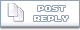# 8 Queens on A ChessboardPlacing 8 Queens on a chessboard is a classic problem and is one of the good software interview question. Following code is my take on this problem. Though instead of starting queen placement at predefined position (at 0,0 or at a corner), 1st queen placement in first row can be specified by user. Discussion/Comments are welcome, please click here to go to discussion forum.

An fun webpage is created with CGI/PERL script where above algorithm can be seen in work. Please go here to experiment with the algorithm.

This program is iterative rule based algorithm. Implementation is mainly C with few C++ extensions (Can be assumed C mainly).

A two dimensional array of 8×8 is used to represent chess board. Value '0' in an element of this array represents that corresponding location in chessboard is empty. Similarly value '99' indicates that corresponding location has a queen placed in it. Any other value in an element indicates influence weight of various queens on this element.

C snippet may look like following

 Chessboad Representation #include #include // Initial Chess Board is empty indicated by 0s at all // locations char chess_board = { {0, 0, 0, 0, 0, 0, 0, 0},                            {0, 0, 0, 0, 0, 0, 0, 0},                            {0, 0, 0, 0, 0, 0, 0, 0},                            {0, 0, 0, 0, 0, 0, 0, 0},                            {0, 0, 0, 0, 0, 0, 0, 0},                            {0, 0, 0, 0, 0, 0, 0, 0},                            {0, 0, 0, 0, 0, 0, 0, 0},                            {0, 0, 0, 0, 0, 0, 0, 0} }; // Place a Queen at location X,Y on Chess Board // Arguments are X,Y coordinates //    Returns 1 if successful int place_a_queen (int x, int y) {    // Limit Verification    if ((x > 7) || (x < 0)) {      printf ("Error!! X coordinates out of range.. \n");      return 0;    }    if ((y > 7) || (y < 0)) {      printf ("Error!! Y coordinates out of range.. \n");      return 0;    }    // Queen can be placed where there is no queen and    // Location is not influenced by any other queen    if (chess_board[x][y] != 0) return 0;    // Queen Positions are indicated by 99 & affected positions are    // indicated by 1    chess_board[x][y] = 99;    // Vertical Row Filling    for (int i=0; i < 8; i++) {      if (i != y) chess_board[x][i] += 1;    }         // Horizontal Row Filling    for (int i=0; i < 8; i++) {      if (i != x) chess_board[i][y] += 1;    }    // Diagonal  Filling    for (int i=x+1, j=y+1; (i<8)&&(j<8); i++, j++)      chess_board[i][j] += 1;         for (int i=x-1, j=y-1; (i>=0)&&(j>=0); i–, j–)      chess_board[i][j] += 1;         for (int i=x+1, j=y-1; (i<8)&&(j>=0); i++, j–)      chess_board[i][j] += 1;    for (int i=x-1, j=y+1; (i>=0)&&(j<8); j++, i–)      chess_board[i][j] += 1;    return 1; } // Arguments are X,Y coordinates //    Returns 1 if successful int pick_a_queen (int x, int y) {    if ((x > 7) || (x < 0)) {      printf ("Error!! X coordinates out of range.. \n");      return 0;    }    if ((y > 7) || (y < 0)) {      printf ("Error!! Y coordinates out of range.. \n");      return 0;    }    if (chess_board[x][y] != 99) return 0;    // Queen Positions are indicated by 2 & affected positions are    // indicated by 1    chess_board[x][y] = 0;    // Vertical Row Filling    for (int i=0; i < 8; i++) {      if (i != y) chess_board[x][i] -= 1;    }         // Horizontal Row Filling    for (int i=0; i < 8; i++) {      if (i != x) chess_board[i][y] -= 1;    }    // Diagonal  Filling    for (int i=x+1, j=y+1; (i<8)&&(j<8); i++, j++)      chess_board[i][j] -= 1;         for (int i=x-1, j=y-1; (i>=0)&&(j>=0); i–, j–)      chess_board[i][j] -= 1;         for (int i=x+1, j=y-1; (i<8)&&(j>=0); i++, j–)      chess_board[i][j] -= 1;    for (int i=x-1, j=y+1; (i>=0)&&(j<8); j++, i–)      chess_board[i][j] -= 1;    return 1; }

Next: Recursive Placement of 8 Queens